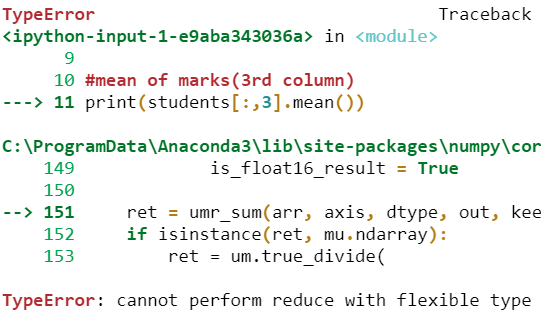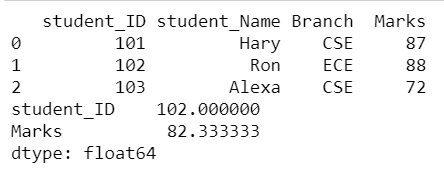Open In App

# How to Fix: TypeError: cannot perform reduce with flexible type

In this article we will discuss TypeError: cannot perform reduce with flexible type and how can we fix it. This error may occur when we find the mean for a two-dimensional NumPy array which consists of data of multiple types.

Dataset in use:

When we create this table using NumPy then this 2D Array consists of data with multiple types. In this, we had String and Integer datatypes. To find the mean value for any of the numeric columns like Marks, it throws TypeError, because it doesn’t know how to take mean when not all the values are numbers (i.e. Student Name, Branch consists data of type string).

Example: Error producing code

## Python3

 `# import necessary packages``import` `numpy as np`` ` `# create a 2D Array``students ``=` `np.array([[``'Student ID'``, ``'Student Name'``, ``'Branch'``, ``'Marks'``],``                     ``[``101``, ``'Hary'``, ``'CSE'``, ``87``],``                     ``[``102``, ``'Ron'``, ``'ECE'``, ``88``],``                     ``[``103``, ``'Alexa'``, ``'CSE'``, ``72``]])`` ` `# mean of marks(3rd column)``print``(students[:, ``3``].mean())`

Output:To overcome this problem create a 2D array using Pandas DataFrame instead of NumPy. Since DataFrame has an index value for each row and name for each column, it helps the interpreter to distinguish between columns of different types.

This single alternative fixes the issue efficiently.

Example: Fixed code

## Python3

 `# import necessary packages``import` `pandas as pd`` ` `# create dataframe``students ``=` `pd.DataFrame({``'student_ID'``: [``101``, ``102``, ``103``],``                         ``'student_Name'``: [``'Hary'``, ``'Ron'``, ``'Alexa'``],``                         ``'Branch'``: [``'CSE'``, ``'ECE'``, ``'CSE'``],``                         ``'Marks'``: [``87``, ``88``, ``72``]})``# Table``print``(students)`` ` `# mean values for all numeric columns``print``(students.mean())`

Output:Students Table and Mean Values Results

In the above example, dataframe mean value is generated for all columns with numeric type if the column name is not specified- student_ID and Marks columns are of type float. So it calculates the mean for those 2 columns and the rest of the columns are of type string. So it won’t calculate the mean value.